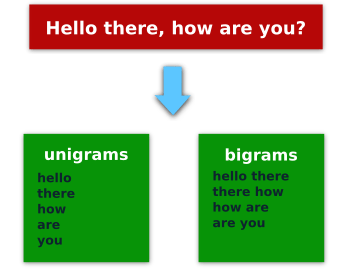# Ruby NLP: N-gram Analysis For Fun & Profit

What would you do if you are given a big collection of text & you want to extract some meaning out of it?

A good start is to break up your text into n-grams.

Here’s a description:

In the fields of computational linguistics and probability, an n-gram is a contiguous sequence of n items from a given sequence of text. – Wikipedia

For example:

If we take the phrase “Hello there, how are you?” then the unigrams (ngrams of one element) would be: `"Hello", "there", "how", "are", "you"`, and the bigrams (ngrams of two elements): `["Hello", "there"], ["there", "how"], ["how", "are"], ["are", "you"]`.

If you learn better with images here is a picture of that:Now let’s see how you can implement this in Ruby!

Before we can get our hands dirty we will need some sample data.

If you don’t have any to work with you could download a few Wikipedia or blog articles. In this particular case, I decided to download some IRC logs from #ruby freenode’s channel.

The logs can be found here:

irclog.whitequark.org/ruby

A note on data formats:

If a plain text version of the resource you want to analyze is not available, then you can use Nokogiri to parse the page and extract the data.

The irc logs are available in plain text by appending `.txt` at the end of the URL so we will take advantage of that.

```require 'restclient'

class LogParser
LOG_DIR  = 'irc_logs'

def initialize(date)
@date = date
@log_name = "#{LOG_DIR}/irc-log-#{@date}.txt"
end

return log_contents if File.exist? @log_name
RestClient.get(url).body
end

def save_page(page)
File.open(@log_name, "w+") { |f| f.puts page }
end

def log_contents
end

def get_messages
save_page(page)
page
end
end

log = LogParser.new("2015-04-15")
msg = log.get_messages
```

This is a pretty straightforward class.

We use RestClient as our HTTP client and then we save the results in a file so we don’t have to request them multiple times while we make modifications to our program.

## Analyzing The Data

Now that we have our data we can analyze it.

Here is a simple Ngram class.

In this class we use the Array#each_cons method which produces the ngrams.

Because this method returns an `Enumerator` we need to call to_a on it to get an `Array`.

```class Ngram
def initialize(input)
@input = input
end

def ngrams(n)
@input.split.each_cons(n).to_a
end
end
```

Then we put everything together using a loop, `Hash#merge!` & `Enumerable#sort_by`.

Like this:

```# Filter words that appear less times than this
MIN_REPETITIONS = 20

total = {}

# Get the logs for the first 15 days of the month and return the bigrams
(1..15).each do |n|
day = '%02d' % [n]
total.merge!(get_trigrams_for_date "2015-04-#{day}") { |k, old, new| old + new }
end

# Sort in descending order
total = total.sort_by { |k, v| -v }.reject { |k, v| v < MIN_REPETITIONS }

total.each { |k, v| puts "#{v} => #{k}" }
```

Note: the `get_trigrams_for_date` method is not here for brevity, but you can find it on github.

This is what the output looks like:

```112 => i want to
82  => is there a
71  => you want to
66  => i don't know
66  => i have a
65  => i need to
```

As you can see wanting to do things is very popular in #ruby 🙂